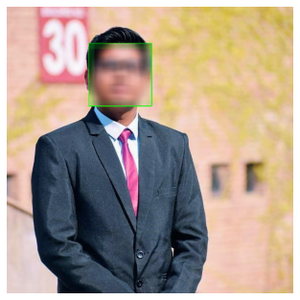# How to Blur Faces in Images using OpenCV in Python?

Prerequisite: OpenCV

OpenCV is a huge open-source library for computer vision, machine learning, and image processing.  It can process images and videos to identify objects, faces, or even the handwriting of a human. When it is integrated with various libraries, such as Numpy which is a highly optimized library for numerical operations, then the number of weapons increases in your Arsenal i.e whatever operations one can do in Numpy can be combined with OpenCV.

This article discusses how a face in an image can be blurred using OpenCV.

Requirements:

Apart from OpenCV module, to obtain this functionality we also need Haar Cascade frontal-face classifier needs to be downloaded. It is available as XML file and is used for detecting faces in an image

Approach

• Import module
• Reading an image using OpenCV
• Plotting it
• Detect face
• Draw a rectangle on the detected face
• Blur the rectangle
• Display output

Below is the implementation.

Input image:Original: my_img.jpg

## Python3

 `# Importing libraries ` `import` `numpy as np ` `import` `cv2 ` `import` `matplotlib.pyplot as plt ` ` `  `# A function for plotting the images ` ` `  ` `  `def` `plotImages(img): ` `    ``plt.imshow(img, cmap``=``"gray"``) ` `    ``plt.axis(``'off'``) ` `    ``plt.style.use(``'seaborn'``) ` `    ``plt.show() ` ` `  ` `  `# Reading an image using OpenCV ` `# OpenCV reads images by default in BGR format ` `image ``=` `cv2.imread(``'my_img.jpg'``) ` ` `  `# Converting BGR image into a RGB image ` `image ``=` `cv2.cvtColor(image, cv2.COLOR_BGR2RGB) ` ` `  `# plotting the original image ` `plotImages(image) ` ` `  `face_detect ``=` `cv2.CascadeClassifier(``'haarcascade_frontalface_alt.xml'``) ` `face_data ``=` `face_detect.detectMultiScale(image, ``1.3``, ``5``) ` ` `  `# Draw rectangle around the faces which is our region of interest (ROI) ` `for` `(x, y, w, h) ``in` `face_data: ` `    ``cv2.rectangle(image, (x, y), (x ``+` `w, y ``+` `h), (``0``, ``255``, ``0``), ``2``) ` `    ``roi ``=` `image[y:y``+``h, x:x``+``w] ` `    ``# applying a gaussian blur over this new rectangle area ` `    ``roi ``=` `cv2.GaussianBlur(roi, (``23``, ``23``), ``30``) ` `    ``# impose this blurred image on original image to get final image ` `    ``image[y:y``+``roi.shape[``0``], x:x``+``roi.shape[``1``]] ``=` `roi ` ` `  ` `  `# Display the output ` `plotImages(image) `

Output:Blurred image

Whether you're preparing for your first job interview or aiming to upskill in this ever-evolving tech landscape, GeeksforGeeks Courses are your key to success. We provide top-quality content at affordable prices, all geared towards accelerating your growth in a time-bound manner. Join the millions we've already empowered, and we're here to do the same for you. Don't miss out - check it out now!

Previous
Next